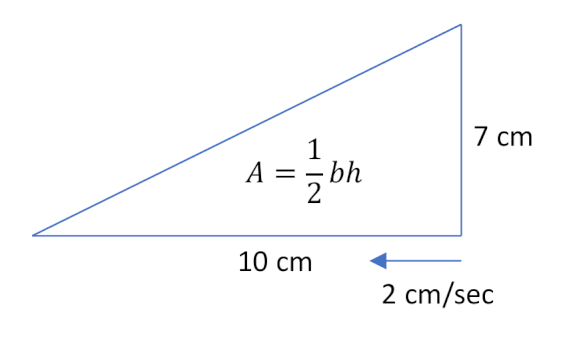$\newenvironment {prompt}{}{} \newcommand {\ungraded }{} \newcommand {\HyperFirstAtBeginDocument }{\AtBeginDocument }$

A right triangle has one leg of constant length 7 cm as shown in the diagram below. The area of the triangle is given by the formula $A=\dfrac {1}{2} b h$, where $b$ is the length of the base of the triangle and $h$ is the height of the triangle. In order to determine how fast its is area changing at the instant that the other leg has length 10 cm, which of the following formulas would you use?$\dfrac {dA}{dt} = \dfrac {dA}{dh} * \dfrac {dh}{dt}$ $\dfrac {dA}{dt} = \dfrac {dA}{db} * \dfrac {db}{dt}$ $\dfrac {dA}{dh} = \dfrac {1}{2}b$ $\dfrac {dA}{db} = \dfrac {1}{2}h$ $\dfrac {dA}{dt} = \dfrac {1}{2}$
$0.002+750$ degrees/minute $0.002*750$ degrees/minute $\dfrac {750}{0.002}$ degrees/minute $\dfrac {0.002}{750}$ degrees/minute $0.002-750$ degrees/minute $750-0.002$ degrees/minute You need to know the current altitude of the plane and the current temperature to figure out the answer.
A square’s side length, $x$, is increasing at a rate of 3 inches per second, as shown below. How quickly is its area increasing?$9$ in$^2$/sec $9x$ in$^2$/sec $6x$ in$^2$/sec $6$ in$^2$/sec $1.5$ in$^2$/sec $1.5x$ in$^2$/sec